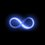# Exponential equations

$2^x-3^{x/2}-1=0$

We can guess or use W|A to figure that $x=2$. But can we crack this problem analytically?

This problem arose out of Karim Mohamed's solution to Big Problem, small solution.Note by John M.
6 years, 8 months ago

This discussion board is a place to discuss our Daily Challenges and the math and science related to those challenges. Explanations are more than just a solution — they should explain the steps and thinking strategies that you used to obtain the solution. Comments should further the discussion of math and science.

When posting on Brilliant:

• Use the emojis to react to an explanation, whether you're congratulating a job well done , or just really confused .
• Ask specific questions about the challenge or the steps in somebody's explanation. Well-posed questions can add a lot to the discussion, but posting "I don't understand!" doesn't help anyone.
• Try to contribute something new to the discussion, whether it is an extension, generalization or other idea related to the challenge.

MarkdownAppears as
*italics* or _italics_ italics
**bold** or __bold__ bold
- bulleted- list
• bulleted
• list
1. numbered2. list
1. numbered
2. list
Note: you must add a full line of space before and after lists for them to show up correctly
paragraph 1paragraph 2

paragraph 1

paragraph 2

[example link](https://brilliant.org)example link
> This is a quote
This is a quote
    # I indented these lines
# 4 spaces, and now they show
# up as a code block.

print "hello world"
# I indented these lines
# 4 spaces, and now they show
# up as a code block.

print "hello world"
MathAppears as
Remember to wrap math in $$ ... $$ or $ ... $ to ensure proper formatting.
2 \times 3 $2 \times 3$
2^{34} $2^{34}$
a_{i-1} $a_{i-1}$
\frac{2}{3} $\frac{2}{3}$
\sqrt{2} $\sqrt{2}$
\sum_{i=1}^3 $\sum_{i=1}^3$
\sin \theta $\sin \theta$
\boxed{123} $\boxed{123}$

Sort by:

Do you mean finding a general solution in terms of functions?(logarithms for example) or just approximating and finding number of solutions????

- 6 years, 8 months ago

Well, general solution and a method of solving... Is there one? Like, you know, $ax^2+bx+c=0 \Rightarrow x=\frac{-b\pm\sqrt{b^2-4ac}}{2a}$, and so on. Does this form of equations have a general solution? If not, that's ok. Just a solution to $2^x-3^{x/2}-1=0$ would be fine too.

Thanks

- 6 years, 8 months ago

A non-rigorous proof would be to note how

$2^x-3^{x/2}$

grows larger and larger as $x$ increases. A quick table would quickly confirm. If we let $y=2^x-3^{x/2}$, we have:

$(2, 1)$,

$(4, 5)$,

$(6, 37)$,

$(8, 175)$,

and so on and so forth.

- 6 years, 8 months ago

Not seeking for a proof here, but an analytical solution. What if we had instead

$2.71828^x-6.67384^{x/2}-360=0$?

Try making tables now.

- 6 years, 8 months ago

Can this even be done? Are equations in form $a^x+b^{x/2}+c=0$ solvable? Seems tempting to complete the square, until we realize it doesn't work like that on exponentials.

Oh, maths.

Thanks, Finn.

- 6 years, 8 months ago